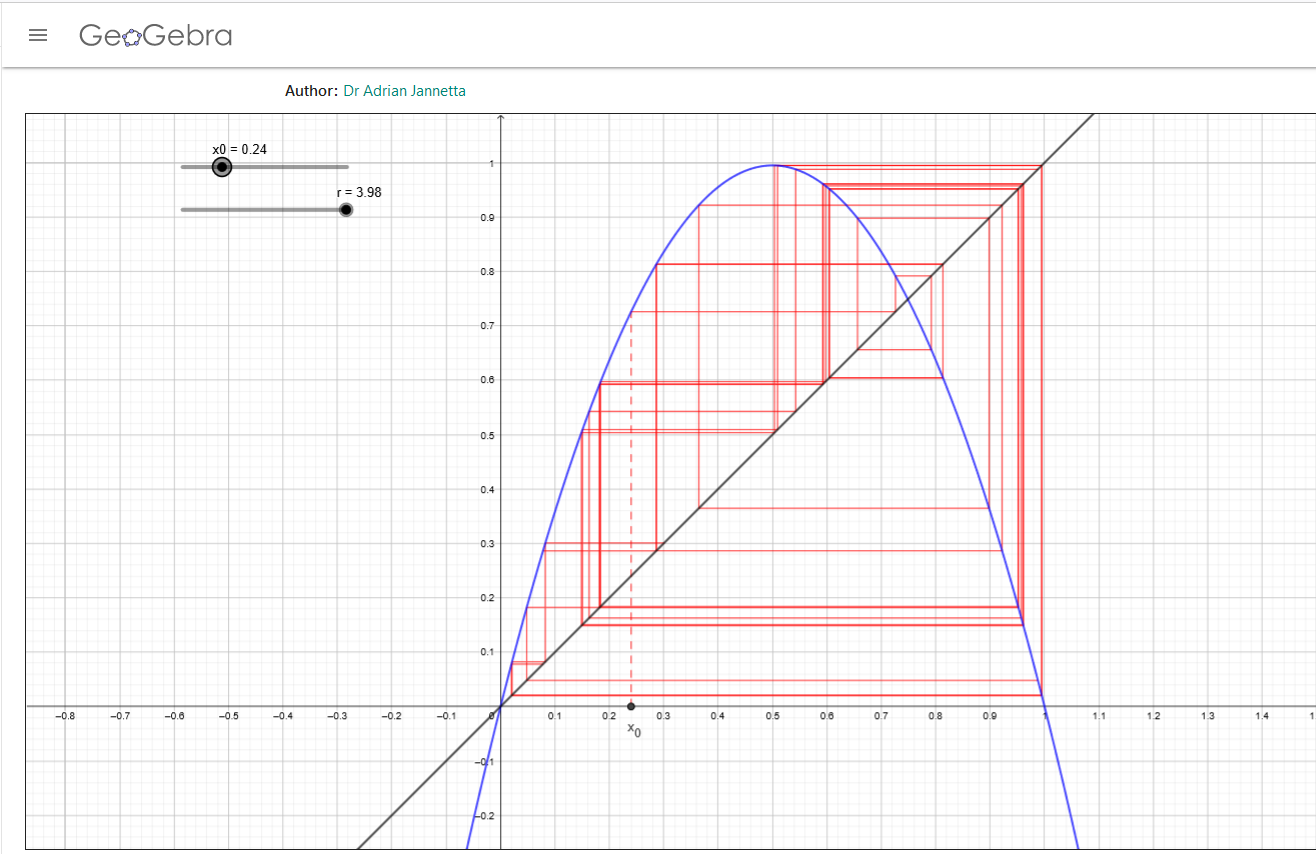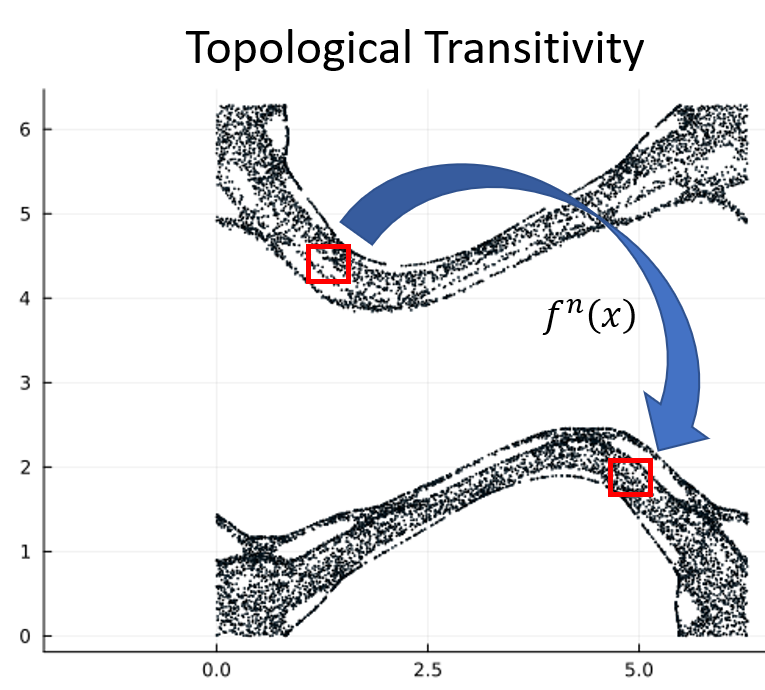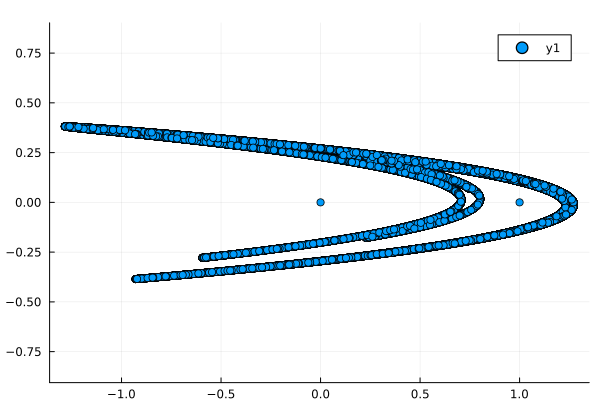# Tools of Chaos

Subtitle: Orbits“No matter where you go, there you are.”
Buckaroo Banzai

In chaos theory, an orbit is the sequence generated by repeatedly applying a function $f$ to the initial point $x_0$.

$S(x_0) = \{x_0,x_1,x_2, \ldots \} = \{x_0, f(x_0),f(f(x_0)), \ldots \}$

## # Pluto Orbits

The previous post, Easy Chaos with Pluto showed how to run a Pluto notebook in Julia. A more in-depth introduction is on the MIT open course Introduction to Computational Thinking which describes the first-time setup of Julia and Pluto and provides a Cheatsheets page.

From JuliaDynamics we’ll use ChaosTools, DynamicalSystems, InteractiveDynamics, and DrWatson. You should also add DifferentialEquations, Plots, and GLMakie with Pkg, the Julia package manager.

## # The Discrete and the Continuous

Chaotic orbits may be either discrete or continuous. In the previous post Easy Chaos with Pluto the logistic function

$x_{n+1} = rx_n(1-x_n)$

is an example of a discrete system. For $x_0 = 0.2$ and $r = 4$, this formula gives $x_1 = 0.64$. The sequence is $\{0.2, 0.64, 0.9216, 0.289, \ldots \}$ which makes discrete steps between iterations.Continuous functions are often written in terms of time, such as the Van der Pol equation

\begin{aligned} \dot{x} &= y \\ \dot{y} &= \mu (1-x^2) y \end{aligned}

Pick any point $P = (x,y)$. The notation $\dot{x}$ and $\dot{y}$ are the derivatives of $x$ and $y$ which tells you how fast point $P$ is moving. Suppose $P = (1,3)$. Putting those coordinates into the Van der Pol equation gives

\begin{aligned} \dot{x} &= 3 \\ \dot{y} &= \mu(1 - 1^2) \times 3 = 0 \end{aligned}

which says that the point $P$ is moving to the right with velocity $3$, and the $y$ velocity is zero. The time variable $t$ doesn’t seem to show up, but $(\dot{x},\dot{y}$) is the velocity of $P$ which is a function of time. In some cases, you may see the notation $\dot{x} = \frac{dx}{dt}$ or $\dot{y} = \frac{dy}{dt}$ indicating that velocities are functions of time. The ratio gives the slope $m$ of the velocity,

$\frac{\dot{y}}{\dot{x}} = \frac{dy}{dt} / \frac{dx}{dt}.$

Starting at point $P_0$, the velocity is $v = (\dot{x},\dot{y})$, so for some small time step $\Delta t$ the next position would be $P_1 = P_0 + \Delta t (\dot{x},\dot{y})$. The time step can be as small as you like making the trajectory, or orbit, of $P_0$ a continuous function.

It isn’t too difficult to generate the sequence of points in the orbit of the logistic function, but for the Van der Pol equation the velocity changes with every small time step, so keeping track of the orbit points becomes tedious. Fortunately, computers can handle these kinds of equations and plot the orbits for us.

## # Chaotic Orbits

Orbits are chaotic if they have the following three properties:

• Sensitive dependence on initial conditions
• Topological transitivity
• Dense periodic orbits

One way to think about these properties is to imagine a car race. At the start they all have slightly different initial conditions. Some engines will be stronger than others, some drivers better than others, and only one car gets the pole position. Each car occupies a slightly different position on the track. These slight differences are the sensitive dependence on initial conditions that will determine the outcome of the race.

When the race starts the cars move down the track and begin to separate, each one completing laps at slightly different times than the others, and the paths that they take may be similar, but each car creates its own path. After enough time, the leaders will begin to lap the stragglers, and even though the cars separated at the start, they’re now back together, which is a little like topological transitivity.

The mathematical definition of topological transitivity says that for any two regions $U$ and $V$ in the orbit, there is some positive integer $n$ such that some of the iterated points in $U$, or $f^n(U)$ can be found in $V$. This holds no matter how small the regions $U$ and $V$ are, and no matter where they are in the orbit.

Not all of the points in $U$ will map into $V$, and not all of the points in $V$ will be the result of iterated points in $U$, but there will be at least one point that does map. Another way to think about this is that every point in the orbit approaches every other point through iterations of $f$.

A consequence of topological transitivity is that an orbit is never two or more independent orbits. Even if the orbit appears to have distinct branches, eventually points in one branch will iterate into every other branch.A periodic orbit is one where $f^n(x_0) = x_0$, so $x_0$ comes back to itself after $n$ iterations of $f$. The cars go around the track and return to the points where they started. The analogy with race cars isn’t quite accurate because the lateral position on the track changes from lap to lap, but maybe you can imagine the propagation of points to be a little like a car race. Dense periodic orbits mean that at any point in the orbit of one point, you can always find the orbit of another point nearby.

These three properties of chaos were first described by Robert L. Devaney in his book, An Introduction to Chaotic Dynamical Systems. A popular non-mathematical book, Chaos: Making a New Science was published by James Gleick in 1987. Wolfram MathWorld says this about the definition of chaos,

Gleick notes that “No one [of the chaos scientists he interviewed] could quite agree on [a definition of] the word itself,” and so instead gives descriptions from a number of practitioners in the field. For example, he quotes Philip Holmes (apparently defining “chaotic”) as, “The complicated aperiodic attracting orbits of certain, usually low-dimensional dynamical systems.” Similarly, he quotes Bai-Lin Hao describing chaos (roughly) as “a kind of order without periodicity.”

Chaotic systems are deterministic which means there is no random component to an orbit. Given a starting position, in principle, you can calculate exactly where a point will be $n$ steps into the future for any value of $n$. What makes them seem random is that two nearby starting points evolve in unpredictable ways.

## # The Orbits Notebook

The Orbits.jl notebook lets you experiment with both discrete and continuous dynamical systems using both built-in equations and systems you can write yourself. Using a built-in method is as simple as loading the dynamical system rule:

ds_henon = Systems.henon()

generating the trajectory

traj_henon = trajectory(ds_henon,100000)

and plotting the result

plot(traj_henon[:,1],traj_henon[:,2], seriestype = :scatter, aspect_ratio = :equal)The equations for the Hénon Map are

\begin{aligned} x_{n+1} &= 1 - \alpha x_n^2 + y_n \\ y_{n+1} &= \beta x_n \end{aligned}

which could have been written in Julia as

function henon_rule(u, p, n)  x, y = u # Current state  α, β = p # Parameters  xn = 1 - α*x^2 + y  yn = β*x  return SVector(xn, yn)end

After setting the initial condition $u$ and the parameter vector $p$, we could generate the trajectory and plot it just as we did with the built-in version. The rule requires a step counter $n$ for discrete systems, or a time $t$ for continuous systems even if these variables don’t show up in the equations.

The Orbits.jl notebook is fully documented so you should be able to change parameters and extend equations or even create new systems. Have fun experimenting!

#### # Image credits

Buckaroo Bonzai: Buckaroo Bonzai, Writeups.org

Orbits.jl - A Pluto notebook to study chaos

## # Software

### # Julia

The Julia Project as a whole is about bringing usable, scalable technical computing to a greater audience: allowing scientists and researchers to use computation more rapidly and effectively; letting businesses do harder and more interesting analyses more easily and cheaply.

Posts using Julia

### # Pluto.jl

Pluto is an environment to work with the Julia programming language. Easy to use like Python, fast like C.

Posts using Pluto.jl

### # DrWatson

DrWatson is a scientific project assistant software. It helps people manage their scientific projects (or any project for that matter). It is a Julia package created to help people increase the consistency of their scientific projects, navigate them and share them faster and easier, manage scripts, existing simulations as well as project source code. DrWatson helps establishing reproducibility, and in general it makes managing a scientific project a simple job.

See all software used on wildpeaches →